# 基于数据优化的保险客户承保预测Insurance Customer Purchase Prediction Based on Data Optimization

DOI: 10.12677/SA.2019.85089, PDF, HTML, XML, 下载: 518  浏览: 2,279  科研立项经费支持

Abstract: In recent years, with the general improvement of people's living standards, the insurance industry ushered in a new spring. The extensive business model has been unable to meet the requirements of the increasing development of insurance companies. How to get rid of the traditional way of mar-keting, quickly discover valuable customers and keep up with the market, is becoming more and more important for insurance companies. This article uses customer data from a life insurance company. Firstly, descriptive statistical analysis was conducted based on the given basic information of customers, call information, insurance information and risk donation information, etc., to view the data situation, and data cleaning was carried out to improve the data quality. Secondly, a separate logistic regression model is used for learning to generate a feasibility analysis report. Then, the combined model of decision tree and logistic regression and the combined model of random forest and logistic regression were respectively used for prediction. Finally, a comparison of the three models shows that the combined model of random forest and logistic regression is more effective.

1. 绪论

1.1. 研究背景及意义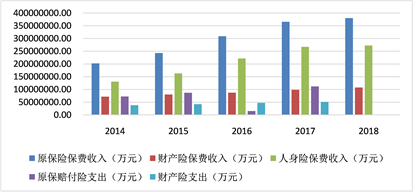Figure 1. Insurance premium income status chart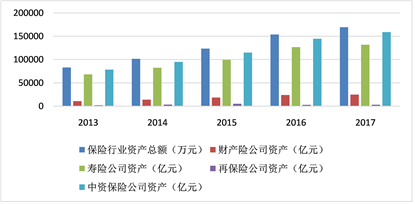Figure 2. Asset position chart of insurance industry

1.2. 国内外研究现状简介

1.3. 研究框架

2. 相关方法介绍

2.1. 决策树模型

2.1.1. 模型简介

2.1.2. 决策树的生成过程：

a) 给定训练集样本；

b) 寻找众多属性特征中使得分类不纯度降低最大或分类纯度增加最大的特征；

c) 根据该属性对数据集进行分类；

d) 不断重复b,c过程生成决策树。如图3即为决策树的样式：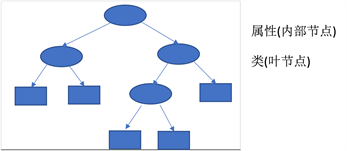Figure 3. Decision tree generation diagram

2.1.3. 决策树的优缺点

1) 决策树思想简单易于理解，容易提取规则；

2) 可用于处理具有缺失属性的样本；

3) 可用于处理不相关特征且运行速度较快。

1) 容易产生过拟合，泛化能力较弱；

2) 容易忽略数据中属性间的关联性；

3) 如果类别太多，决策树会错分的情况会增加的比较快。

2.2. 随机森林模型

2.2.1. bagging简介

2.2.2. RF简介

2.2.3. 随机森林的构建过程：

a) 从训练集样本中进行bootstrap抽样，生成n个子集；

b) 将这n个子集分别作为训练集训练处多棵决策树；

c) 运用测试集进行预测输出，对每棵决策树使用简单投票法获得分类结果。如图4即为随机森林的构建过程：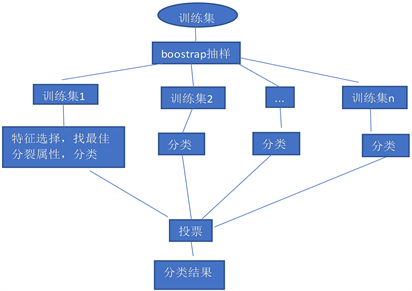Figure 4. Random forest generation diagram

2.2.4. 随机森林的优缺点

1) 能够很好的解决过拟合问题，抗噪性强，在数据集上表现良好；

2) 对数据集的适应力强，能够处理高维数据；

3) 既能处理离散型数据也能处理连续性数据；

4) 思想清晰，便于理解。

1) 对数据量少，数据维度低的数据效果不一定好；

2) 执行速度相比于boosting模型快，但相对于决策树模型较慢；

3) 在某些噪音较大的分类或回归问题上会过拟合。

2.3. 逻辑回归模型

2.3.1. 逻辑斯谛分布

$F\left(x\right)=P\left(X\le x\right)=\frac{1}{1+{\text{e}}^{-\left(x-u\right)/\gamma }}$

$f\left(x\right)={F}^{\prime }\left(x\right)=\frac{{\text{e}}^{-\left(x-u\right)/\gamma }}{\gamma {\left(1+{\text{e}}^{-\left(x-u\right)/\gamma }\right)}^{2}}$

2.3.2. 逻辑斯谛回归模型

$P\left(Y=1|x\right)=\frac{\text{exp}\left(w\cdot x+b\right)}{1+\text{exp}\left(w\cdot x+b\right)}$

$P\left(Y=0|x\right)=\frac{1}{1+\text{exp}\left(w\cdot x+b\right)}$

$P\left(Y=1|x\right)=\pi \left(x\right)$$P\left(Y=0|x\right)=1-\pi \left(x\right)$

$y=\frac{1}{1+{\text{e}}^{-wx}}$

2.3.3. 模型的优缺点

1) 逻辑回归模型最大的优点是模型极具可解读性  ；

2) 训练速度快；

3) 占用内存小。

1) 准确率不够高；

2) 数据平衡问题较难处理；

3) 无法筛选特征。

2.4. 评估指标

2.4.1. Accuracy

AUC (Area Under Curve)值指的是ROC曲线下方的面积大小 。ROC曲线一般位于y = x上方，因此AUC的取值范围一般在0.5和1之间 。在对角线之上的区域越大则AUC的值越大。AUC的值越大，分类效果越好，一般而言：

2.4.2. 精确率、召回率和F1值

F1值为精确率和召回率的调和均值，相当于这两个的综合评价指标。

3. 数据集

3.1. 数据介绍

Table 1. Customer data feature fields

3.2. 数据的预处理

1) 异常值处理

2) 缺失值处理

3) 重复数据处理

4) 无效变量剔除

5) 数据平衡处理

3.3. 特征工程

1) 特征组合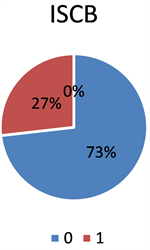Figure 5. Positive and negative sample distribution

2) 分箱处理

3) 数据标准化

4) 离散化

5) 相关性分析

a) 指标与标签之间的相关性分析

b) 指标间的相关性分析

4. 数据建模

4.1. 逻辑回归模型

Accuracy is: 0.907340.

4.2. 基于决策树特征选择的逻辑回归

4.2.1. 决策树特征选择Table 3. Ranking chart of feature importance

4.2.2. 决策树与Logistic模型融合

Accuracy is: 0.915393.

4.3. 基于RF特征选择的逻辑回归

4.3.1. RF特征选择Table 5. Ranking chart of feature importance

4.3.2. RF与Logistic模型融合

Accuracy is: 95.196. [RFC full test]

5. 总结与展望

1) 模型优化研究。本文研究分析所使用的模型依旧是传统的逻辑回归模型，没有创新的提出更新颖的模型，这是后续需要继续关注和研究的方向

2) 本实验中随机森林模型与逻辑回归模型进行组合的效果最高，可能因为随机森林模型是一种集成算法，之后的研究中可以考虑将GBDT，xgboost等流行算法应用到保险数据分析中去。

  苗东. 大都会保险公司客户关系管理研究[D]: [硕士学位论文]. 上海: 华东理工大学, 2013.  卞爱军. 基于信息化平台的寿险客户细分管理研究——以扬州寿险公司为例[D]: [硕士学位论文]. 南京: 南京理工大学, 2008.  柯新喜. 基于决策树模型的社会保险客户分类研究[J]. 福建电脑, 2016, 32(6): 105-107.  王贵龙. 基于关联向量机的保险客户识别研究[D]: [硕士学位论文]. 西安: 西安工业大学, 2011.  赵萍. 数据挖掘在寿险客户关系管理中的应用[D]: [硕士学位论文]. 天津: 天津大学, 2007.  董娜, 常建芳, 吴爱国. 基于贝叶斯模型组合的随机森林预测方法[J]. 湖南大学学报(自然科学版), 2019, 46(2): 123-130.  苏杭西子. 基于随机森林模型的个人信用风险评估研究[D]: [硕士学位论文]. 长沙: 湖南大学, 2018.  李航. 统计学习[M]. 北京: 清华大学出版社, 2012:77-79.  邴欣. 机器学习在推荐系统中的应用[D]: [硕士学位论文]. 济南: 山东大学, 2016.  钱超. 基于特征优化的逻辑回归模型在广告点击率问题中的应用研究[D]: [硕士学位论文]. 武汉: 华中师范大学, 2018.  宋天龙. Python数据分析与数据化运营[M]. 北京: 机械工业出版社, 2017: 99-102.  刘晨晨. 基于数据挖掘的通信客户流失预警模型研究[D]: [硕士学位论文]. 武汉: 华中师范大学, 2017.  王文敬. 基于SMOTE过抽样法的个人信用评分模型研究[D]: [硕士学位论文]. 上海: 上海师范大学, 2019.